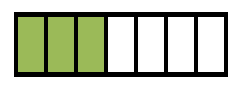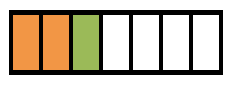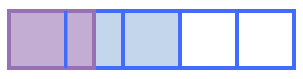### Home > CC1 > Chapter 5 > Lesson 5.1.3 > Problem5-25

5-25.

Calculate each of the following parts of parts.

1. $\frac { 2 } { 3 } \text { of } \frac { 3 } { 7 }$

• Draw a diagram to represent three sevenths. A rectangle divided into 7 equal vertical parts, with the first 3 parts shaded.

• Since the green shaded region is three pieces, you can see that two thirds of this region would be the orange shaded region. The first 2 parts change from being shaded green to being shaded orange.

• $\frac{2}{7}$1. $\quad \frac { 1 } { 2 } \text { of } \frac { 3 } { 5 }$

• At right is a diagram representing one half of three fifths. Use it to help you find the answer to this problem.